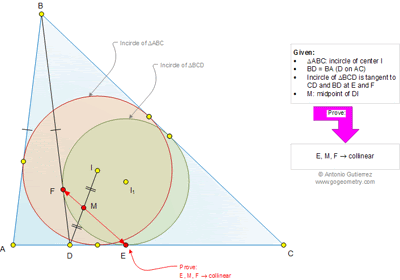## Saturday, March 8, 2014

### Geometry Problem 991: Triangle, Incircle, Tangent Points, Isosceles, Midpoint, Collinearity, Congruence, Circle

Level: Mathematics Education, High School, Honors Geometry, College.

Click the figure below to view the complete problem 991#### 1 comment:

1.Let altitude BK of triangle ABC cut EF at N and AC at K
let incircle I tangent to AC at L
Let p and p’ are haft perimeter of triangles BDC and ABC
Using properties of incircles I , I1 and isosceles triangle ABD we have LE= p’-p => LE=AK=KD
We also have ∠(DEF)=1/2∠(FDA)= ∠(IAL) and AL=KE
Triangles ALI congruent to EKN => KLIN is a rectangle
Since DE=KL=NI => DEIN is a parallelogram and diagonals will bisect each other.